So far, we have covered HMM-GMM acoustic modeling and some practical issues related to context and less frequent phonemes.

# Introduction to Neural Network acoustic modeling

There is an alternative way to build an acoustic model, and it consists in using neural networks to:

• take an input acoustic frame
• and output a score for each phone

Let’s consider a single-layer neural network, that takes as an input an acoustic frame $$X_t$$ and outputs phonetic scores $$f(t)$$ (one score for each phone).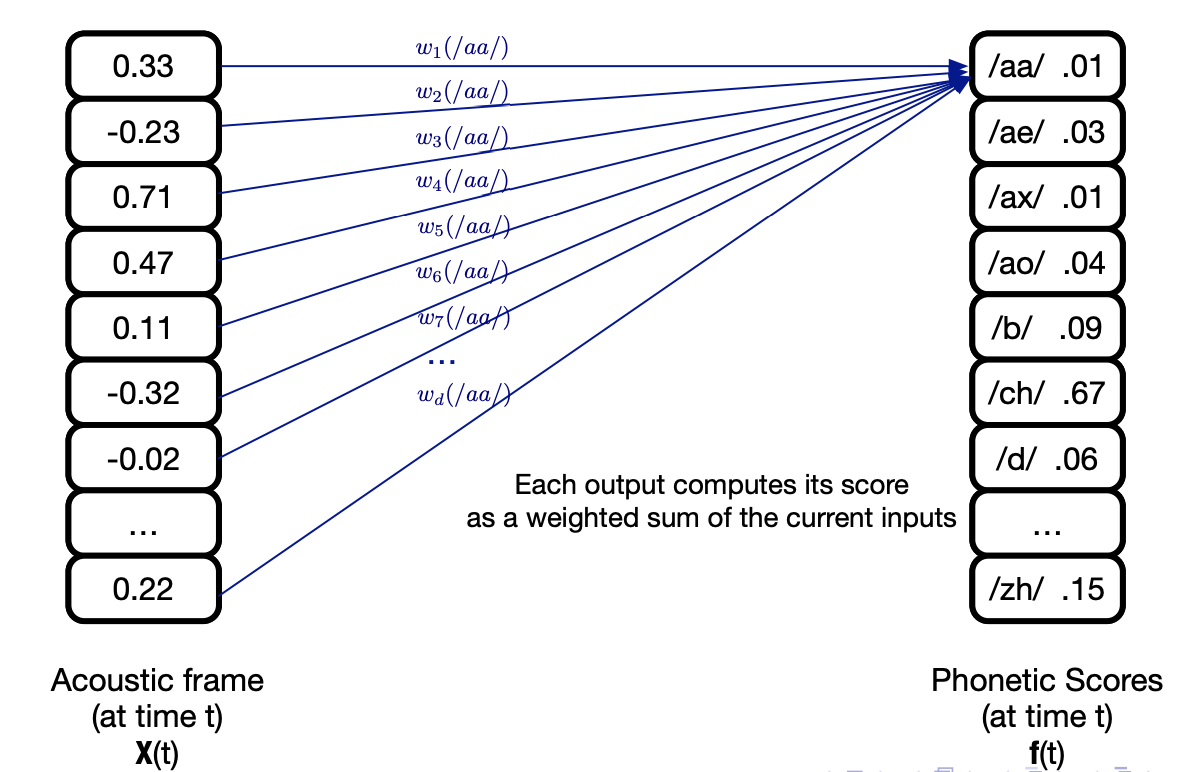It can be expressed as:

$f = Wx + b$

Where $$W$$ is the weight matrix made of weights $$w_{ij}$$ that reflect the weifht between input $$i$$ and output $$j$$, and $$b$$ is the bias term.

How do we learn the parameters $$W$$ and $$b$$? We target the minimization of the error function $$E$$, the Mean Square Error (MSE) between the output and the target:

$E = 0.5 \times \frac{1}{T} \sum_{t=1}^T {\mid \mid f(x_t) - r(t) \mid \mid}^2$

Where $$r(t)$$ are the target outputs.

The error minimization is typically done using gradient descent, and we must compute the terms:

$$\frac{d E}{d W}$$ and $$\frac{d E}{d b}$$

In SGD, we:

• intialize weights and biases with small random numbers
• randomise the order of training data example
• then for each epoch:
• take a minibact
• compute network outputs
• backpropagate and update the weights

The network that predicts phonetic scores is a classifier, so we need to take a softmax to force output values to act as probabilities:

$y_j = \frac{exp(f(x_j))}{\sum_{k=1}^K exp(f(x_k))}$

Where $$f(x_j) = \sum_{d=1}^D w_{jd} x_d + b_j$$

However, the MSE is not the wisest choice when working with probabilities. We can directly maximize the log probability of observing the correct label using the Cross-Entropy (CE) error function:

$E_t = - \sum{j=1}^J r_j^t \ln y_j^t$

Using CE, the gradients of the outputs weights simplify to:

$\frac{dE^t}{dW_{jd}} = (y_j^t - r_j^t) x_d$

There are several extensions possible to this very very simple model:

• use more context by taking multiple frames into account
• add more hidden layers to obtains DNNs
• use activation functions such as ReLU

This looks interesting, and overall simpler than the HMM-GMM. But there is a major limitation, since we cannot do speech recognition with this approach. There are several phone recognition tasks:

• frame classification: classify each frame of data
• phone classification: classify each segment of data (what Neural Networks can do)
• phone recongition: segment the data and label the segment

Using only DNN, we lack the notion of data segmentation that HMMs are good at doing. But can’t we mix HMMs and DNNs ?

# HMM-DNN acoustic modeling

In an HMM-GMM, replacing the GMM by a DNN to estimate output pdfs build a so-called HMM-DNN architecture. In a HMM-DNN, we consider one-state per phone, and train a NN as a phone-state classifier.

It can be shown that the outputs corresponding to class $$j$$ given an input $$x_t$$ are an estimate of the posterior probability $$P(q_t = j \mid x_t)$$, $$q_t$$ being a state, because we have softmax outputs and use a CE loss function.

And using Bayes Rule, we can relate the posterior $$P(q_t = j \mid x_t)$$ to the likelihood $$P(x_t \mid q_t = j)$$:

$P(q_t \mid x_t) = \frac{P(x_t \mid q_t = j) P(q_t = j)}{P(X_t)}$

If we want HMM-DNNs to output probabilities, we should scale the likelihoods:

$\frac{P(q_t = j \mid x_t)}{P(q_t = j)} = \frac{P(x_t \mid q_t = j)}{P(x_t)}$

This means we can obtain scaled likelihoods by dividing each network output by the prior, i.e. the relative frequency of class $$j$$ in training data.

There are several approaches to continuous speech recognition with HMM-DNN:

• 1 state per phone (each NN can output typically 60 classes if 60 phone classes)
• 3 state context-independent (CI) models, where each phone has 3 states, modeled by 3 NNs
• State-clustered context-dependent (CD) models, 1 NN output per tied state

The architecture of a HMM-DNN is presented below: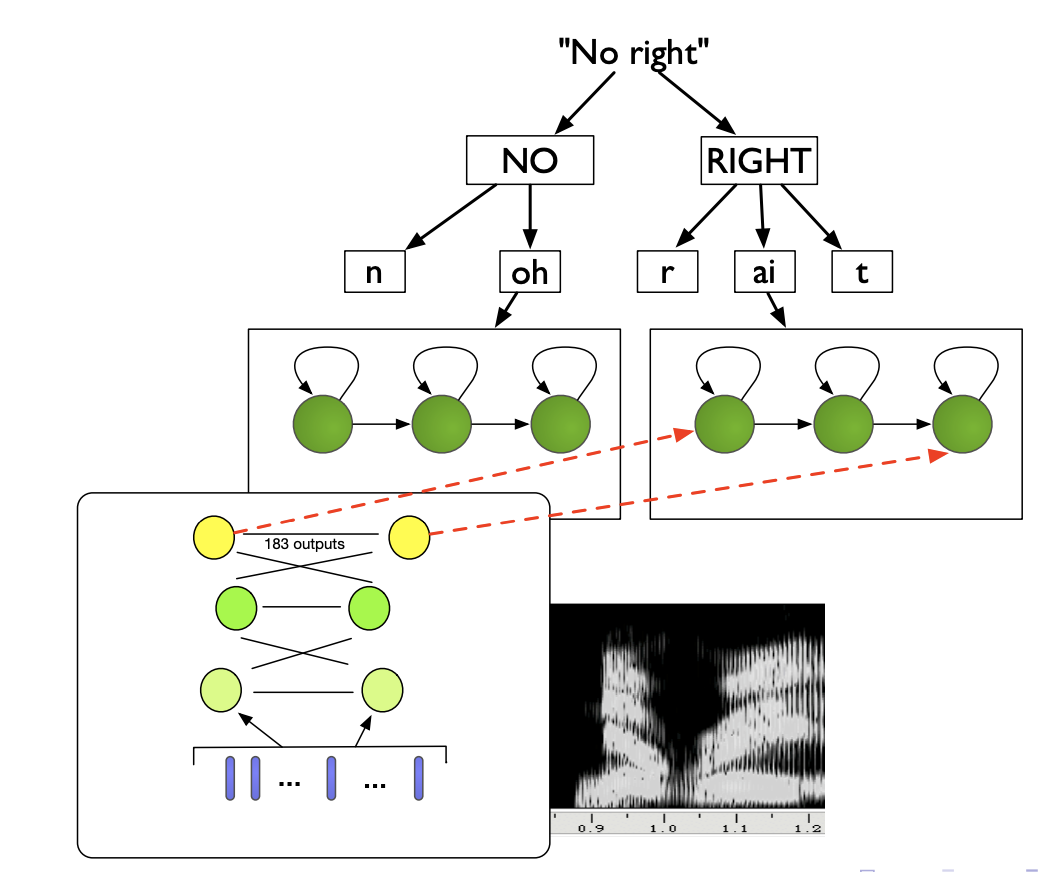NN are more flexible, learn richer representations and handle correlated features. In terms of speech features, experiments indicate that mel-scaled filter bank features (FBANK) work better than MFCCs, and results in better clustering when applying t-SNE on the hidden layers. Indeed, in FBANK the useful information is distributed over all the features, whereas in MFCC it is concentrated in the first few.

# Modelling phonetic context with DNNs

Modling the phonetic context with DNNs was considered hard until 2011, but a simple solution emerged: use the state-tying process from a GMM system, and train a HMM-DNN on it.

More precisely, the context-dependent hybrid HMM/DNN approach is:

• Train a GMM/GMM system on your data
• Use a phonetic decision tree to determine the HMM tied states for infrequent states
• Perform Viterbi alignment using the trained HMM/GMM and the training data
• Train a neural network to map the input speech features to a label representing a context-dependent tied HMM-state, instead of contenxt-independent phones
• this increases the number of possible labels, and each frame is labelled with Viterbi aligned tied states
• we then train the NN using gradient descent

Concretely, we model the acoustic context by including neighbour frames in the input layer.

We use richer Neural Network models for acoustic context:

• Recurrent Neural Networks (RNNs)
• Time-delay Neural Networks (TDNNs)

## Time-delay Neural Networks

In TDNNs, higher hidden layers take input from a larger acoustic context, and lower hidden layers from narrower contexts. TDNNs can be seen as a 1D convolutional network.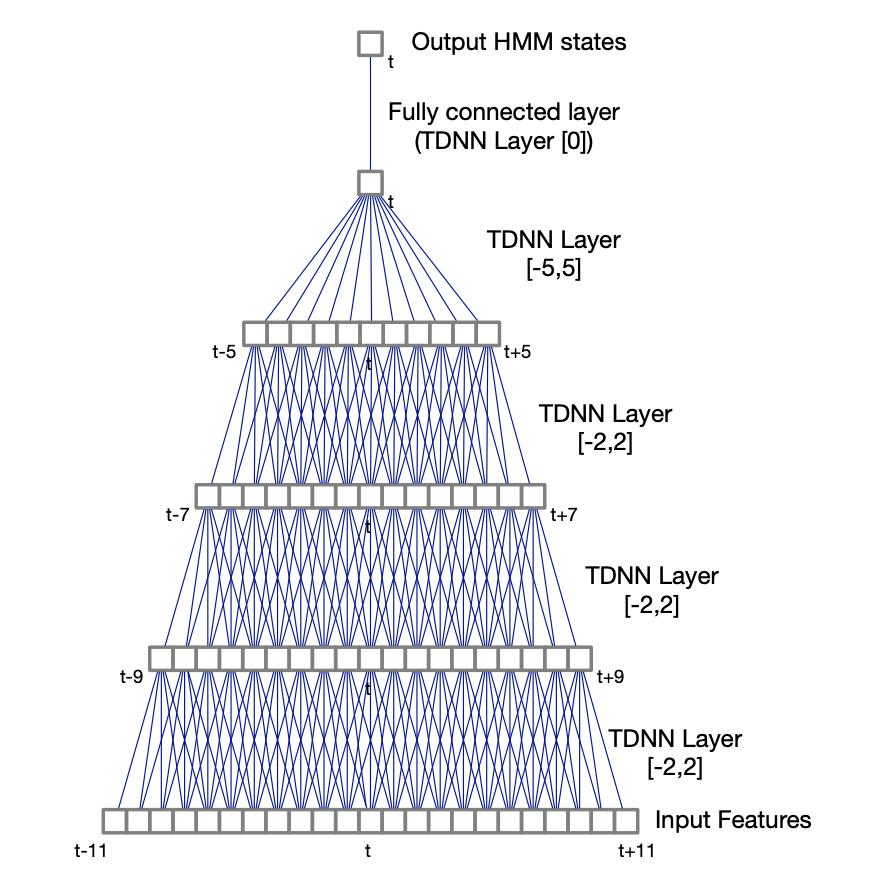A TDNN with a context (-2, 2) has 5 times more weights than a regular DNN. For this reason, sub-sampled TDNNs are explored. Due to the large overlaps between input contexts at adjacent time steps, which are likely to be correlated, we take a sub-sample window of hidden unit activations. It reduces computation time and model weights.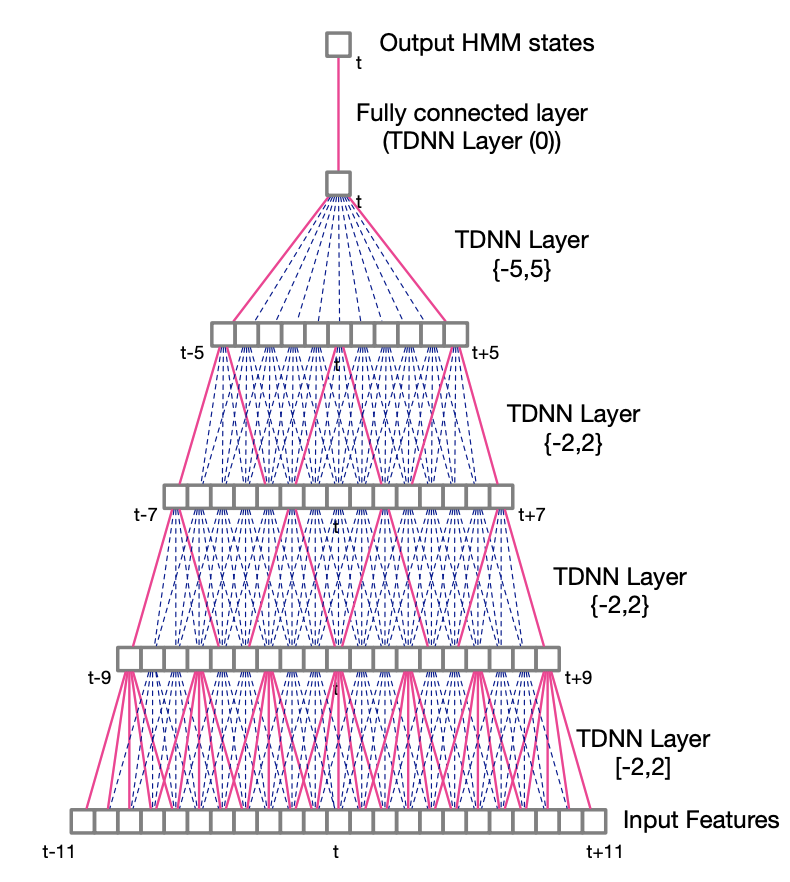## Recurrent Neural Networks

In its unfolded representation, the RNN for a sequence of T inputs is a T-layer network with shared weights. It can keep information through time.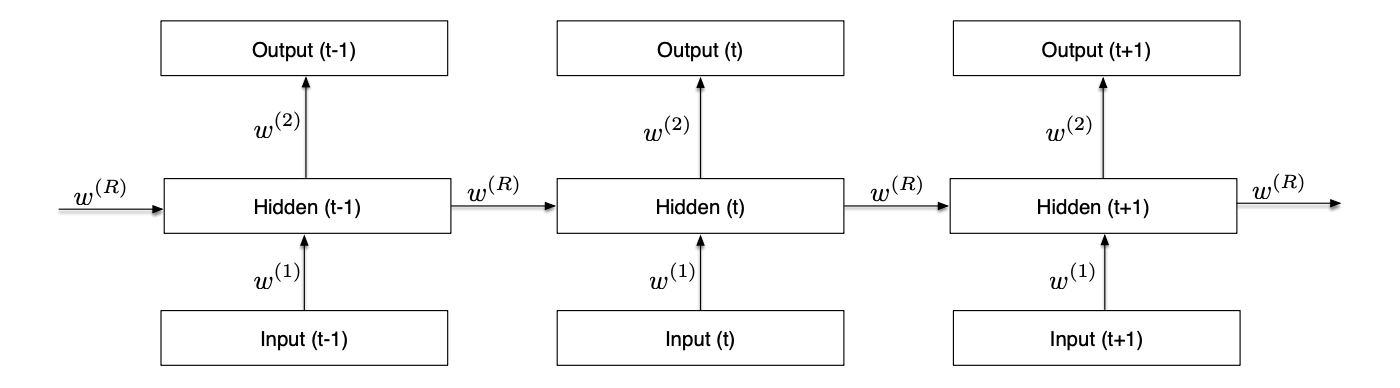LSTMs avoid the vanishing gradient problem of RNNs. Bidirectional RNNs consider both the right and the left context, in a forward layer and a backward layer. Deep RNNs have several hidden layers, and deep bidirectional LSTM combine the advantages of all these methods: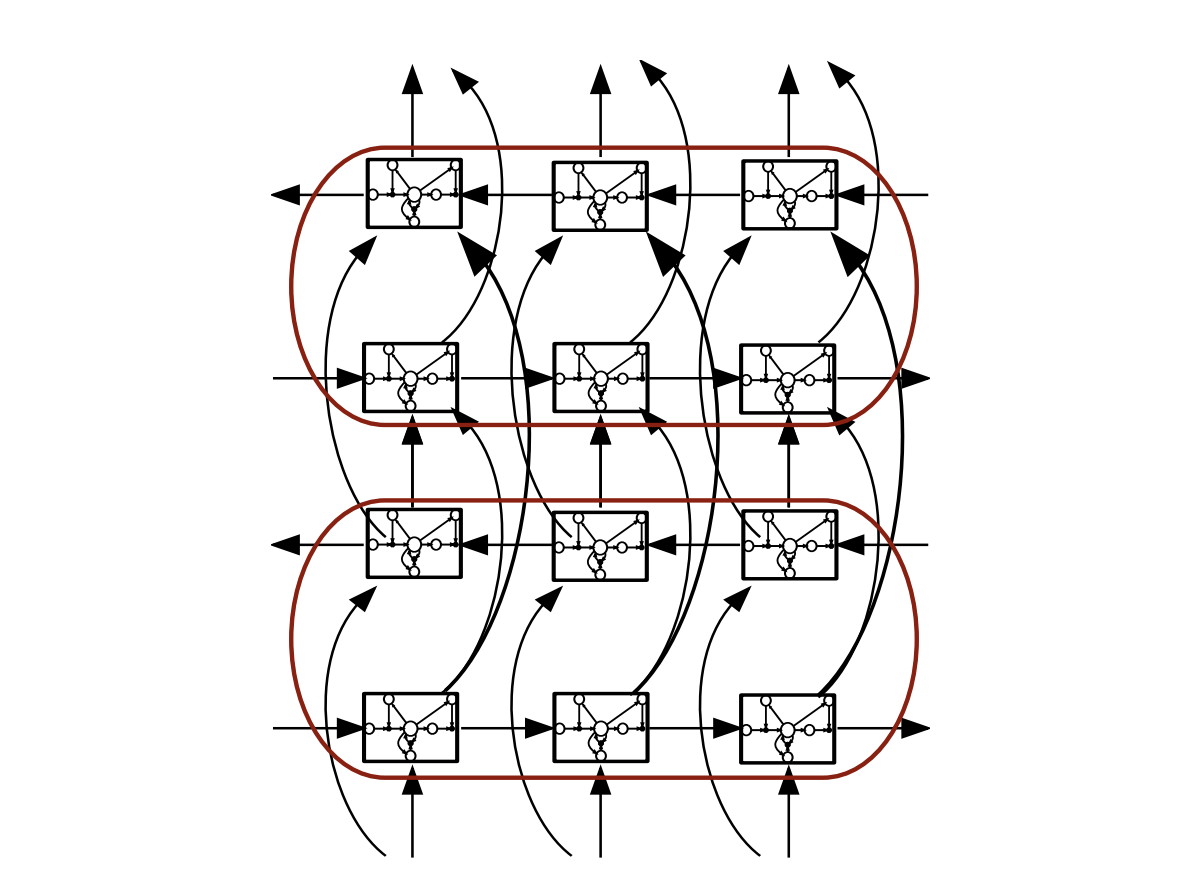Here is an example of a Bidirectional LSTM Acoustic Model training on the Switchboard dataset:

• LSTM has 4-6 bidirectional layers with 1024 cells/layer (512 each direction)
• 256 unit linear bottleneck layer
• 32k context-dependent state outputs
• Input features:
• 40-dimension linearly transformed MFCCs (plus ivector)
• 64-dimension log mel filter bank features (plus first and second derivatives)
• concatenation of MFCC and FBANK features
• Training: 14 passes frame-level cross-entropy training, 1 pass sequence training (2 weeks on a K80 GPU)

LSTMs + feature fusion currently reach close to state-of-the-art.